# 3.4e: Exercises - Polynomial Graphs

$$\newcommand{\vecs}{\overset { \rightharpoonup} {\mathbf{#1}} }$$ $$\newcommand{\vecd}{\overset{-\!-\!\rightharpoonup}{\vphantom{a}\smash {#1}}}$$$$\newcommand{\id}{\mathrm{id}}$$ $$\newcommand{\Span}{\mathrm{span}}$$ $$\newcommand{\kernel}{\mathrm{null}\,}$$ $$\newcommand{\range}{\mathrm{range}\,}$$ $$\newcommand{\RealPart}{\mathrm{Re}}$$ $$\newcommand{\ImaginaryPart}{\mathrm{Im}}$$ $$\newcommand{\Argument}{\mathrm{Arg}}$$ $$\newcommand{\norm}{\| #1 \|}$$ $$\newcommand{\inner}{\langle #1, #2 \rangle}$$ $$\newcommand{\Span}{\mathrm{span}}$$ $$\newcommand{\id}{\mathrm{id}}$$ $$\newcommand{\Span}{\mathrm{span}}$$ $$\newcommand{\kernel}{\mathrm{null}\,}$$ $$\newcommand{\range}{\mathrm{range}\,}$$ $$\newcommand{\RealPart}{\mathrm{Re}}$$ $$\newcommand{\ImaginaryPart}{\mathrm{Im}}$$ $$\newcommand{\Argument}{\mathrm{Arg}}$$ $$\newcommand{\norm}{\| #1 \|}$$ $$\newcommand{\inner}{\langle #1, #2 \rangle}$$ $$\newcommand{\Span}{\mathrm{span}}$$$$\newcommand{\AA}{\unicode[.8,0]{x212B}}$$

### A: Concepts

Exercise $$\PageIndex{A}$$

1) What is the difference between an $$x$$-intercept and a zero of a polynomial function $$f$$?

2) If a polynomial function of degree $$n$$ has $$n$$ distinct zeros, what do you know about the graph of the function?

3) What is the relationship between the degree of a polynomial function and the maximum number of turning points in its graph? .

4) Explain how the factored form of the polynomial helps us in graphing it.

5) If the graph of a polynomial just touches the $$x$$-axis and then changes direction, what can we conclude about the factored form of the polynomial?

1. The $$x$$-intercept is where the graph of the function crosses the $$x$$-axis, and the zero of the function is the input value for which $$f(x)=0$$.

3. The maximum number of turning points is one less than the degree of the polynomial.

5. There will be a factor raised to an even power.

### B: Multiplicity from an Equation

Exercise $$\PageIndex{B}$$

$$\bigstar$$ Find the zeros and give the multiplicity of each.

 6) $$f(x)=(x+2)^3(x−3)^2$$ 7) $$f(x)=x^2(2x+3)^5(x−4)^2$$ 8) $$f(x)=x^3(x−1)^3(x+2)$$ 9) $$f(x)=x^2(x^2+4x+4)$$ 10) $$f(x)=(2x+1)^3(9x^2−6x+1)$$ 11) $$f(x)=(3x+2)^5(x^2−10x+25)$$ 12) $$f(x)=x(4x^2−12x+9)(x^2+8x+16)$$ 13) $$f(x)=x^6−x^5−2x^4$$ 14) $$f(x)=3x^4+6x^3+3x^2$$ 15) $$f(x)=4x^5−12x^4+9x^3$$ 16) $$f(x)=2x^4(x^3−4x^2+4x)$$ 17) $$f(x)=4x^4(9x^4−12x^3+4x^2)$$
 7. $$0$$ and $$4$$ with multiplicity $$2$$,  $$−\dfrac{3}{2}$$ with multiplicity $$5$$ 9. $$0$$ with multiplicity $$2$$, $$-2$$ with multiplicity $$2$$ 11. $$−\dfrac{2}{3}$$ with multiplicity $$5$$, $$5$$ with multiplicity $$2$$ 13. $$0$$ with multiplicity $$4$$,  $$2$$ and $$-1$$ with multiplicity $$1$$ 15. $$\dfrac{3}{2}$$ with multiplicity $$2$$,  $$0$$ with multiplicity $$3$$ 17. $$0$$ with multiplicity $$6$$, $$\dfrac{2}{3}$$ with multiplicity $$2$$

### C: Multiplicity from a Graph

Exercise $$\PageIndex{C}$$

$$\bigstar$$ Use the graph to identify zeros and multiplicity.

 19)20)21)22)19. $$–4, –2, 1, 3$$ with multiplicity $$1$$ 21. $$–2, 3$$ each with multiplicity $$2$$

### D: Graph polynomials

Exercise $$\PageIndex{D}$$

$$\bigstar$$ Graph the polynomial functions. State the $$x$$- and $$y$$- intercepts, multiplicity, and end behavior.

 24) $$f(x)=(x+3)^2(x−2)$$ 25) $$g(x)=(x+4)(x−1)^2$$ 26) $$h(x)=(x−1)^3(x+3)^2$$ 27) $$k(x)=(x−3)^3(x−2)^2$$ 28) $$m(x)=−2x(x−1)(x+3)$$ 29) $$n(x)=−3x(x+2)(x−4)$$ 30. $$a(x) = x(x + 2)^{2}$$ 31. $$g(x) = x(x + 2)^{3}$$ 32. $$f(x) = -2(x-2)^2(x+1)$$ 33. $$g(x) = (2x+1)^2(x-3)$$ 34. $$f(x) = x^{3}(x + 2)^{2}$$ 35. $$P(x) = (x - 1)(x - 2)(x - 3)(x - 4)$$ 36. $$q(x) = (x + 5)^{2}(x - 3)^{4}$$ 37. $$h(x) = x^2(x-2)^2(x+2)^2$$ 38. $$h(t) = (3-t)(t^2+1)$$ 39. $$Z(b) = b(42 - b^{2})$$
 25. $$x$$-intercepts, $$(1, 0)$$ with multiplicity $$2$$, $$(–4, 0)$$ with multiplicity $$1$$, $$y$$- intercept $$(0, 4)$$ . As $$x→−∞$$, $$f(x)→−∞$$, as $$x→∞,$$ $$f(x)→∞$$.27. $$x$$-intercepts $$(3,0)$$ with multiplicity $$3$$, $$(2,0)$$ with multiplicity $$2$$, $$y$$- intercept $$(0,–108).$$ As $$x→−∞,$$ $$f(x)→−∞$$, as $$x→∞,$$ $$f(x)→∞$$.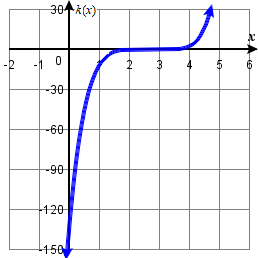29. $$x$$-intercepts $$(0, 0),(–2, 0),(4, 0)$$ with multiplicity $$1$$, $$y$$-intercept (0, 0). As $$x→−∞,$$ $$f(x)→∞$$, as $$x→∞,$$ $$f(x)→−∞$$.31. $$(-2,0)$$ multiplicity $$3$$,       $$(0,0)$$ multiplicity 1,        y-intercept $$(0 ,0 )$$,       end behaviour: $$\nwarrow \dots \nearrow$$33. $$(-\frac{1}{2} ,0 )$$ multiplicity $$2$$,       $$(3 ,0 )$$ multiplicity $$1$$,        y-intercept $$(0 ,-3 )$$,       end behaviour: $$\swarrow \dots \nearrow$$35. $$(1 ,0 )$$,  $$(2 ,0 )$$, $$(3 ,0 )$$, $$(4 ,0 )$$       all multiplicity $$1$$,       y-intercept  $$(0 , 24)$$,       end behaviour: $$\nwarrow \dots \nearrow$$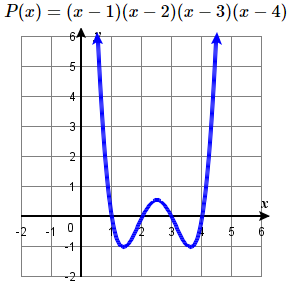37. $$(-2 ,0 )$$, $$(2 ,0 )$$, $$(0 ,0 )$$        all multiplicity $$2$$, y-intercept $$(0 ,0 )$$,       end behaviour: $$\nwarrow \dots \nearrow$$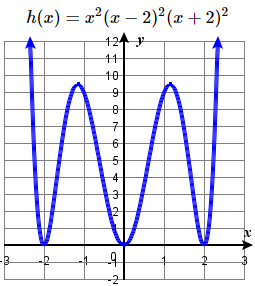39. $$(\sqrt{42} ,0 )$$, $$(-\sqrt{42} ,0 )$$, $$(0 ,0 )$$        all multiplicity $$1$$, y-intercept $$(0 ,0 )$$,        end behaviour: $$\nwarrow \dots \searrow$$$$\bigstar$$ Graph the polynomial functions. State the $$x$$- and $$y$$- intercepts, multiplicity, and end behavior.

 41. $$f\left(x\right)=\left(x+3\right)^{2} (x-2)$$ 42. $$g\left(x\right)=\left(x+4\right)\left(x-1\right)^{2}$$ 43. $$h\left(x\right)=\left(x-1\right)^{3} \left(x+3\right)^{2}$$ 44. $$k\left(x\right)=\left(x-3\right)^{3} \left(x-2\right)^{2}$$  45. $$m\left(x\right)=-2x\left(x-1\right)(x+3)$$ 46. $$n\left(x\right)=-3x\left(x+2\right)(x-4)$$ 47. $$f(x) = 9x - x^3$$ 48. $$f(x) =8 + x^3$$ 49. $$f(x) = x^4 - 25x^2$$ 50. $$f(x) =16 - x^4$$ 51. $$f(x) = -x^4 + 2x^3 + 8x^2$$ 52. $$f(x) =x^3+7x^2 -9x$$ 53. $$f(x) = 2x^3 + 12x^2 - 8x - 48$$ 54. $$f(x) = 4x^4 + 10x^3 - 4x^2 -10x$$
 41. $$(-3 ,0 )$$ multiplicity $$2$$,       $$(2 ,0 )$$ multiplicity $$1$$,        y-intercept$$(0 ,-18 )$$,   $$\swarrow \dots \nearrow$$43. $$( -3, 0)$$ multiplicity $$2$$,       $$(1 , 0)$$ multiplicity $$3$$,       y-intercept$$( 0, -9)$$,  $$\swarrow \dots \nearrow$$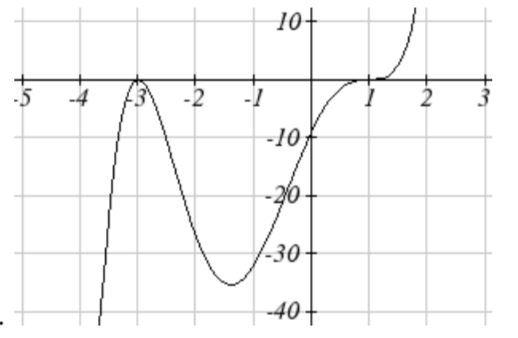45. $$(-3 , 0)$$, $$(0 , 0)$$, $$(1 , 0)$$       all multiplicity $$1$$,        y-intercept$$(0 ,0 )$$, $$\nwarrow \dots \searrow$$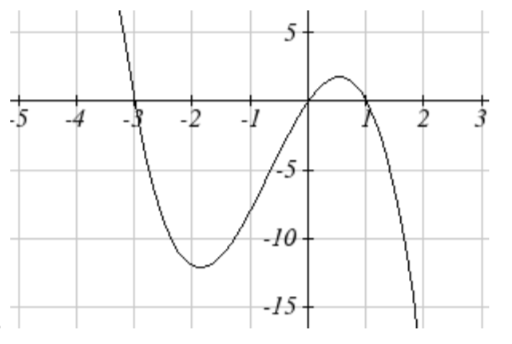47. $$(-3 , 0)$$, $$(0 , 0)$$, $$(3 , 0)$$       all multiplicity $$1$$,        y-intercept $$(0 ,0 )$$, $$\nwarrow \dots \searrow$$49. $$( -5, 0)$$, $$( 5, 0)$$ both multiplicity $$1$$,       $$(0 , 0)$$ multiplicity $$2$$,        y-intercept $$(0 ,0 )$$, $$\nwarrow \dots \nearrow$$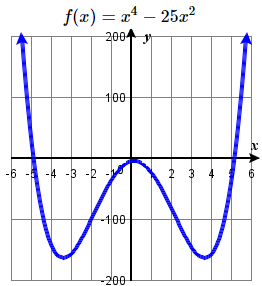51. $$( -2, 0)$$, $$( 4, 0)$$ both multiplicity $$1$$,        $$(0 , 0)$$ multiplicity $$2$$,        y-intercept $$(0 ,0 )$$, $$\swarrow \dots \searrow$$53. $$(-6 , 0)$$, $$(-2 , 0)$$, $$(2 , 0)$$       all multiplicity $$1$$,        y-intercept $$(0 , -48)$$,  $$\swarrow \dots \nearrow$$### E: Polynomial Degree from a Graph

Exercise $$\PageIndex{E}$$

$$\bigstar$$ Determine the least possible degree of the polynomial function shown.

 61)62)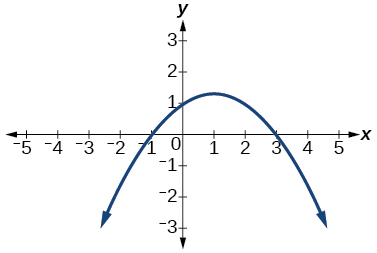63)64)65)66)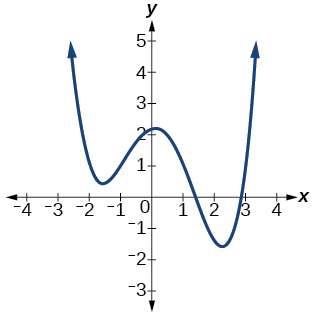67)68)61. $$3$$, $$\qquad$$ 63. $$5$$, $$\qquad$$ 65. $$3$$, $$\qquad$$ 67. $$5$$

### F: Construct an Equation from a graph

Exercise $$\PageIndex{F}$$

$$\bigstar$$ Use the graphs to write the formula for the polynomial function of least degree.

 69)70)71)72)73.74)69. $$f(x) = -(x+3)(x+1)(x-3)$$   or  $$f(x) = -\frac{2}{9}(x+3)(x+1)(x-3)$$
71. $$f(x) = (x+2)^2(x-3)$$   or  $$f(x) = \frac{1}{4}(x+2)^2(x-3)$$
73. $$f(x) = -(x+3)(x+2)(x-2)(x-4)$$   or  $$f(x) = -\frac{1}{24}(x+3)(x+2)(x-2)(x-4)$$

$$\bigstar$$ Use the graphs to write a formula for the polynomial function of least degree.

 75)76)77)78)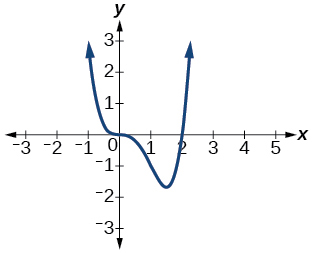79)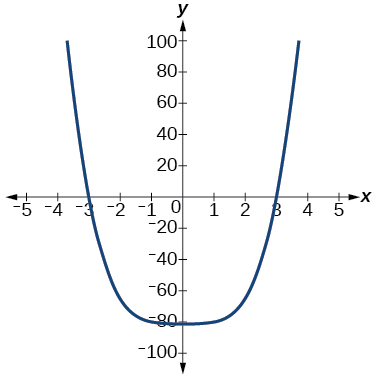80)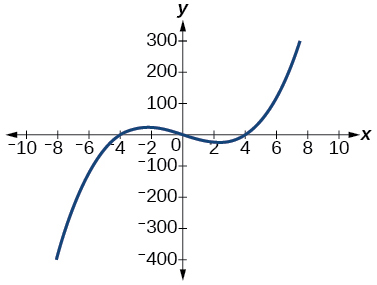81)82)75. $$f(x)=(x−500)^2(x+200)$$    77. $$f(x)=(x+300)^2(x-100)^3$$
79. $$f(x)=(x+3)(x-3)(x^2+10)$$    81. $$f(x)=4x(x-5)(x-7)$$

$$\bigstar$$ Use the graphs to write a formula for the polynomial function of least degree.

 83.84(a).84(b).85.86.87.88.89. .90.83. $$y = \dfrac{1}{24} (x + 4) (x + 2) (x - 3)^2$$ 85. $$y = \dfrac{1}{12} (x + 2)^2 (x - 3)^2$$ 87. $$y = \dfrac{1}{6} (x + 3) (x + 2) (x - 1)^3$$ 89. $$y = -\dfrac{1}{16} (x + 3)(x + 1) (x - 2)^2 (x - 4)$$

### G: Construct an Equation from a Description

Exercise $$\PageIndex{G}$$

$$\bigstar$$ Use the information about the graph of a polynomial function to determine the function. Assume the leading coefficient is $$1$$ or $$-1$$. There may be more than one correct answer.

91) The $$y$$-intercept is $$(0,−4)$$. The $$x$$-intercepts are $$(−2,0), (2,0)$$. Degree is $$2$$. End behavior:   $$\nwarrow \dots \nearrow$$

92) The $$y$$-intercept is $$(0,9)$$. The $$x$$-intercepts are $$(−3,0), (3,0)$$. Degree is $$2$$. End behavior:   $$\swarrow \dots \searrow$$

93) The $$y$$-intercept is $$(0,0)$$. The $$x$$-intercepts are $$(0,0), (2,0)$$. Degree is $$3$$. End behavior:   $$\swarrow \dots \searrow$$

94) The $$y$$-intercept is $$(0,1)$$. The x-intercept is $$(1,0)$$. Degree is $$3$$. End behavior:   $$\nwarrow \dots \searrow$$

95) The $$y$$-intercept is $$(0,1)$$. There is no $$x$$-intercept. Degree is $$4$$. End behavior:   $$\nwarrow \dots \nearrow$$

$$\bigstar$$ Use the given information about the polynomial graph to write the equation.

97) Degree $$3$$. Zeros at $$x=–2$$,$$x=1$$, and $$x=3$$. $$y$$-intercept at $$(0,–4)$$

98) Degree $$3$$. Zeros at $$x=–5$$, $$x=–2$$, and $$x=1$$. $$y$$-intercept at $$(0,6)$$

99) Degree $$5$$. Roots of multiplicity $$2$$ at $$x=3$$ and $$x=1$$. Root of multiplicity $$1$$ at $$x=–3$$.
$$\quad$$$$y$$-intercept at $$(0,9)$$

100) Degree $$4$$. Root of multiplicity $$2$$ at $$x=4$$. Roots of multiplicity $$1$$ at $$x=1$$ and $$x=–2$$.
$$\quad$$$$y$$-intercept at $$(0,–3)$$

101) Degree $$5$$. Double zero at $$x=1$$. Triple zero at $$x=3$$. Passes through the point $$(2,15)$$

102) Degree $$3$$. Zeros at $$x=4$$, $$x=3$$, and $$x=2$$. $$y$$-intercept at $$(0,−24)$$

103) Degree $$3$$. Zeros at $$x=−3$$, $$x=−2$$ and $$x=1$$. $$y$$-intercept at $$(0,12)$$

104) Degree $$5$$. Roots of multiplicity $$2$$ at $$x=−3$$ and $$x=2$$. Root of multiplicity $$1$$ at $$x=−2$$. $$y$$-intercept at $$(0, 4)$$.

105) Degree $$4$$. Roots of multiplicity $$2$$ at $$x=\dfrac{1}{2}$$. Roots of multiplicity $$1$$ at $$x=6$$ and $$x=−2$$. $$y$$-intercept at $$(0,18)$$

106) Double zero at $$x=−3$$. Triple zero at $$x=0$$. Passes through the point $$(1,32)$$.

107. Degree 3. Zeros at $$x$$ = -2, $$x$$ = 1, and $$x$$ = 3. Vertical intercept at (0, -4)

108. Degree 3. Zeros at $$x$$ = -5, $$x$$ = -2, and $$x$$ = 1. Vertical intercept at (0, 6)

109. Degree 5. Roots of multiplicity 2 at $$x$$ = 3 and $$x$$ = 1. Root of multiplicity 1 at $$x$$ = -3. Vertical intercept at (0, 9)

110. Degree 4. Root of multiplicity 2 at $$x$$ = 4. Roots of multiplicity 1 at $$x$$ = 1 and $$x$$ = -2.
$$\quad$$Vertical intercept at (0, -3)

111. Degree 5. Double zero at $$x$$ = 1. Triple zero at $$x$$ = 3. Passes through the point (2, 15)

112. Degree 5. Single zero at $$x$$ = -2 and $$x$$ = 3. Triple zero at $$x$$ = 1. Passes through the point (2, 4)

 91. $$f(x)=x^2−4$$ 93. $$f(x)=x^3−4x^2+4x$$ 95. $$f(x)=x^4+1$$ 97. $$f(x)=−\dfrac{2}{3}(x+2)(x−1)(x−3)$$ 99. $$f(x)=\dfrac{1}{3}(x−3)^2(x−1)^2(x+3)$$ 101. $$f(x) =−15(x−1)^2(x−3)^3$$ 103. (f(x)=−2(x+3)(x+2)(x−1)\) 105. $$f(x)=−\dfrac{3}{2}(2x−1)^2(x−6)(x+2)$$ 107. $$y = -\dfrac{2}{3} (x + 2) (x - 1) (x - 3)$$ 109. $$y = \dfrac{1}{3} (x - 1)^2 (x - 3)^2 (x + 3)$$ 111. $$y = -15(x - 1)^2 (x - 3)^3$$

### H: Turning Points

Exercise $$\PageIndex{H}$$

$$\bigstar$$ Determine whether the graph of the function provided is a graph of a polynomial function. If so, determine the number of turning points and the least possible degree for the function.

 115)116)117)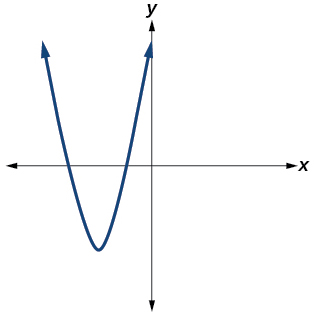118)119)120)121)115. Yes. Number of turning points is $$2$$. Least possible degree is $$3$$.

117. Yes. Number of turning points is $$1$$. Least possible degree is $$2$$.

119. Yes. Number of turning points is $$0$$. Least possible degree is $$1$$.

212. Yes. Number of turning points is $$0$$. Least possible degree is $$1$$.

$$\star$$

3.4e: Exercises - Polynomial Graphs is shared under a not declared license and was authored, remixed, and/or curated by LibreTexts.